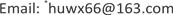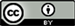﻿ 均以5为尾数的两整数之积的简化算法 A Simplified Algorithm for the Product of Two Integers with a Mantissa of 5

Interdisciplinary Science Letters
Vol. 03  No. 01 ( 2019 ), Article ID: 29280 , 4 pages
10.12677/ISL.2019.31003

A Simplified Algorithm for the Product of Two Integers with a Mantissa of 5

Wenxiang Hu1,2,3*, Xuanping Yang1,4, Zhaoxi Hu1,4

1Jingdong Xianghu Microwave Chemistry Union Laboratory, Beijing Excalibur Space Military Academy of Medical Sciences, Beijing

2School of Chemical Engineering and Pharmacy, Wuhan Institute of Technology, Wuhan Hubei

3Aerospace Systems Division, Strategic Support Troops, Chinese People’s Liberation Army, Beijing

4Beijing Xianghu Science and Technology Development Co., Ltd., BeijingReceived: Feb. 25th, 2019; accepted: Mar. 12th, 2019; published: Mar. 19th, 2019ABSTRACT

This paper establishes a simplified algorithm that multiplies two integers with a single digit of 5, which is especially convenient for oral calculation and quick calculation. It has practical application value in social life.

Keywords:Integer, Multiplier, Single Digit 5, Simplified Algorithm, Popularization and Application

1北京神剑天军医学科学院京东祥鹄微波化学联合实验室，北京

2武汉工程大学化工与制药学院，湖北 武汉

3中国人民解放军战略支援部队航天系统部，北京

4北京祥鹄科技发展有限公司，北京1. 引言

2. 简化算法公式的建立

$n5×n5=\left[n\left(n+1\right)\right]25=\left[n×n+\frac{n+n}{2}\right]25$

$n5×\left(n+1\right)5=\left[n\left(n+1\right)+n\right]75=\left[n×\left(n+1\right)+\frac{n+\left(n+1\right)-1}{2}\right]75$

$n5×\left(n+2\right)5=\left[n×\left(n+2\right)+\frac{n+\left(n+2\right)}{2}\right]25$

$n5×\left(n+3\right)5=\left[n×\left(n+3\right)+\frac{n+\left(n+3\right)-1}{2}\right]75$

$n5×\left(n+4\right)5=\left[n×\left(n+4\right)+\frac{n+\left(n+4\right)}{2}\right]25$

$n5×\left(n+5\right)5=\left[n×\left(n+5\right)+\frac{n+\left(n+4\right)-1}{2}\right]75$

……

$n5×m5=\left[n×m+\frac{n+m}{2}\right]25$ (1)

$n5×m5=\left[n×m+\frac{n+m-1}{2}\right]75$ 当m, n只有一个为奇数 (2)

$n5×m5=\left[n×\left(m+1\right)+\frac{m-n}{2}\right]25$ (3)

$n5×m5=\left[n×\left(m+1\right)+\frac{m-n-1}{2}\right]75$ 当m, n只有一个为奇数 (4)

(3) (4)与(1) (2)完全等价。

(1) (2)式用文字可以表述为： $n5×m5$ 等于m与n的积再加上m + n的平均数，尾跟25，或当m, n

3. 简化算法公式的适用范围及推广应用

3.1. 简化算法公式的适用范围

$45×85=\left[4×8+\frac{4+8}{2}\right]25=3825$

$65×75=\left[6×7+\frac{6+7-1}{2}\right]75=4875$

3.2. 简化算法公式的推广应用

$35×45=35×\left(35+10\right)=35×35+35×10=1575$

$35×95=35×\left(35+60\right)=35×35+35×60=1225+2100=3325$

$35×95=\left[3×9+\frac{3+9}{2}\right]25=3325$

4. 小结

A Simplified Algorithm for the Product of Two Integers with a Mantissa of 5[J]. 交叉科学快报, 2019, 03(01): 11-14. https://doi.org/10.12677/ISL.2019.31003

1. 1. 胡文祥. 简化计算的诀窍[Z]. 大学生学术讨论会, 武汉, 1979, 研究生Seminar报告, 北京, 1982.

2. 2. 千桥飞梦编写组. 千桥飞梦——胡文祥学习研究成果实录[M]. 北京: 知识产权出版社, 2014.

3. 3. 《千桥飞梦》编写组. 千桥飞梦——胡文祥哲学社会科学相关思考录[M]. 第二卷. 武汉: 武汉出版社, 2015.

4. 4. 胡文祥. 社会生物学胡氏约等式[J]. 交叉科学快报, 2017, 1(1): 30-34. https://doi.org/10.12677/isl.2017.11006

5. 5. 胡文祥. 黎曼猜想与H值138的奇妙性[J]. 交叉科学快报, 2019. (In Press)

6. 6. Hu, W.X., Zhao, Z.X. and Liu, M. (2013) How Many Elements Exist in the World? Applied Mechanics and Materials, 328, 1011-1016. https://doi.org/10.4028/www.scientific.net/AMM.328.1011

7. NOTES

*通讯作者。